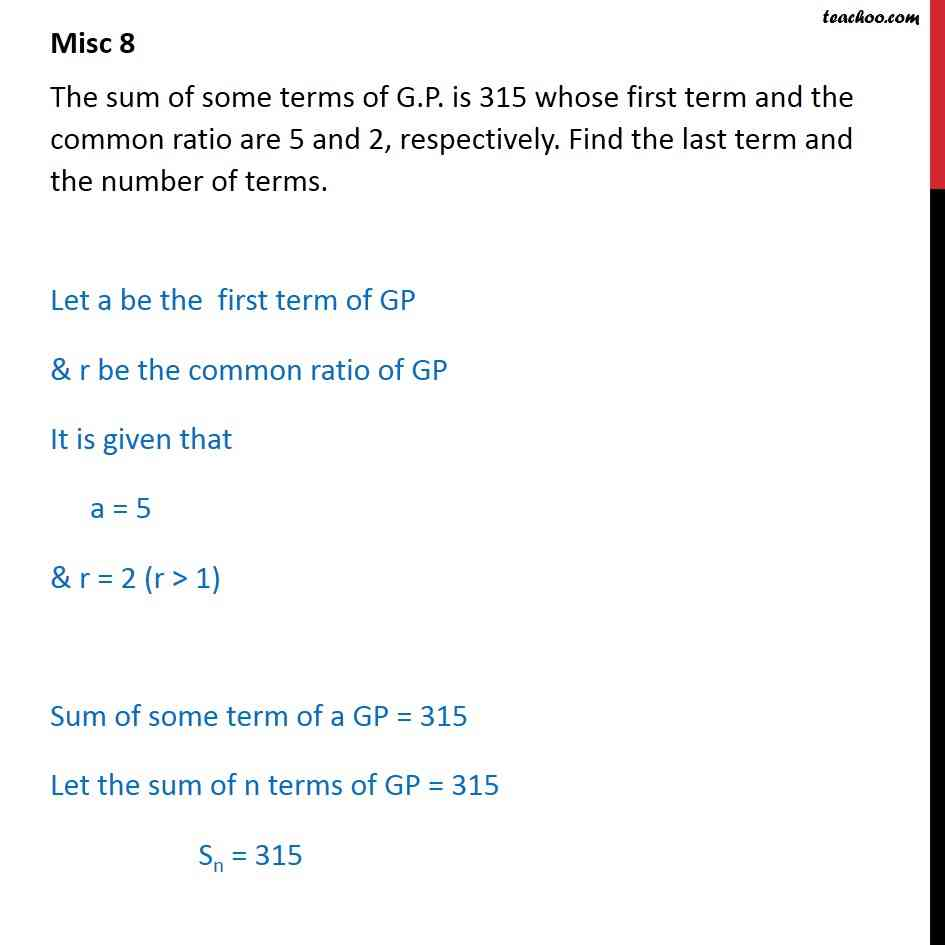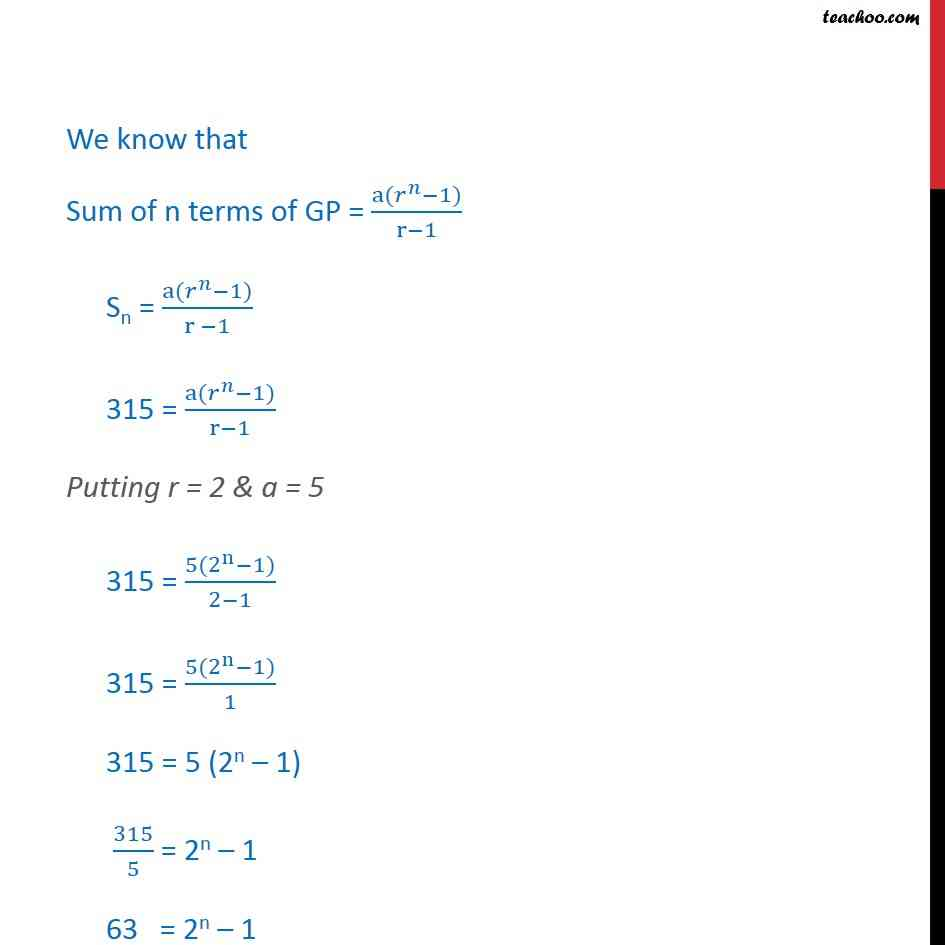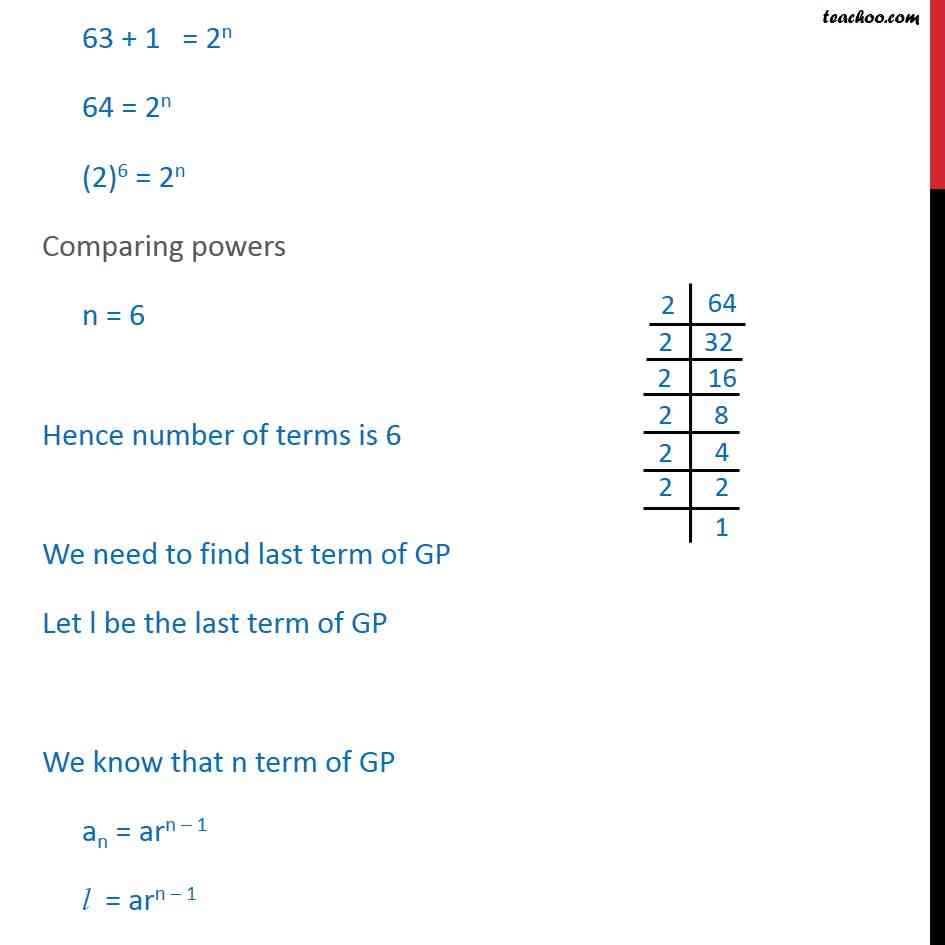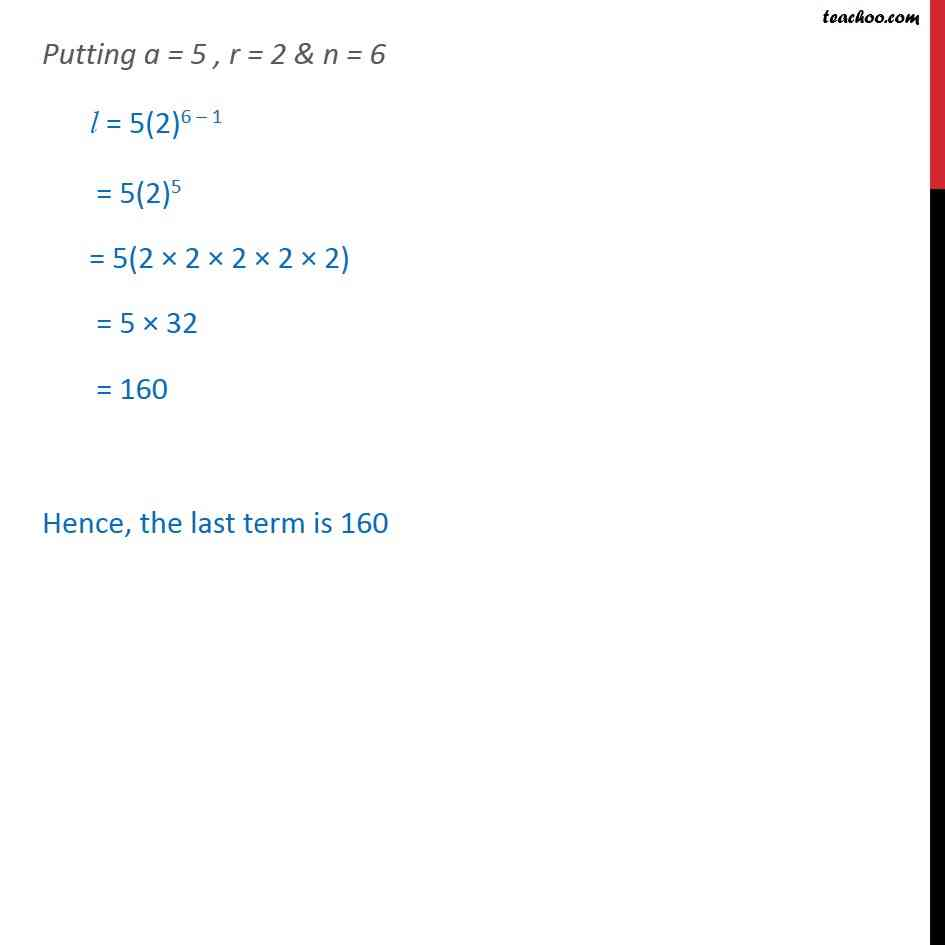Geometric Progression(GP): Formulae based

Chapter 8 Class 11 Sequences and Series
Concept wiseLearn in your speed, with individual attention - Teachoo Maths 1-on-1 Class

### Transcript

Misc 2 The sum of some terms of G.P. is 315 whose first term and the common ratio are 5 and 2, respectively. Find the last term and the number of terms. Let a be the first term of GP & r be the common ratio of GP It is given that a = 5 & r = 2 (r > 1) Sum of some term of a GP = 315 Let the sum of n terms of GP = 315 Sn = 315 We know that Sum of n terms of GP = (a( ^ 1))/(r 1) Sn = (a( ^ 1))/(r 1) 315 = (a( ^ 1))/(r 1) Putting r = 2 & a = 5 315 = (5(2n 1))/(2 1) 315 = (5(2n 1))/1 315 = 5 (2n 1) 315/5 = 2n 1 63 = 2n 1 64 = 2n (2)6 = 2n comparing powers n = 6 Hence number of terms is 6 We need to find last term of GP Let l be the last term of GP We know that n term of GP an = arn 1 l = arn 1 Putting a = 5 , r = 2 & n = 6 l = 5(2)6 1 = 5(2)5 = 5(2 2 2 2 2) = 5 64 = 320 Hence, the last term is 320